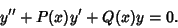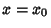## Regular Singular Point

Consider a second-order Ordinary Differential EquationIfandremain Finite at, thenis called an Ordinary Point. If eitherordiverges as, thenis called a singular point. If eitherordiverges asbutandremain Finite as, thenis called a regular singular point (or Nonessential Singularity).

Arfken, G. Singular Points.'' §8.4 in Mathematical Methods for Physicists, 3rd ed. Orlando, FL: Academic Press, pp. 451-453 and 461-463, 1985.